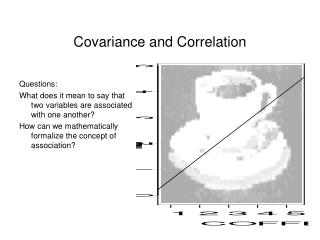DownloadDownload PresentationCovariance and Correlation

# Covariance and Correlation

Télécharger la présentation## Covariance and Correlation

- - - - - - - - - - - - - - - - - - - - - - - - - - - E N D - - - - - - - - - - - - - - - - - - - - - - - - - - -
##### Presentation Transcript

1. Covariance and Correlation Questions: What does it mean to say that two variables are associated with one another? How can we mathematically formalize the concept of association?

2. Limitation of covariance • One limitation of the covariance is that the size of the covariance depends on the variability of the variables. • As a consequence, it can be difficult to evaluate the magnitude of the covariation between two variables. • If the amount of variability is small, then the highest possible value of the covariance will also be small. If there is a large amount of variability, the maximum covariance can be large.

3. Limitations of covariance • Ideally, we would like to evaluate the magnitude of the covariance relative to maximum possible covariance • How can we determine the maximum possible covariance?

4. Go vary with yourself • Let’s first note that, of all the variables a variable may covary with, it will covary with itself most strongly • In fact, the “covariance of a variable with itself” is an alternative way to define variance:

5. Go vary with yourself • Thus, if we were to divide the covariance of a variable with itself by the variance of the variable, we would obtain a value of 1. This will give us a standard for evaluating the magnitude of the covariance. Note: I’ve written the variance of X as sXsX because the variance is the SD squared

6. Go vary with yourself • However, we are interested in evaluating the covariance of a variable with another variable (not with itself), so we must derive a maximum possible covariance for these situations too. • By extension, the covariance between two variables cannot be any greater than the product of the SD’s for the two variables. • Thus, if we divide by sxsy, we can evaluate the magnitude of the covariance relative to 1.

7. Spine-tingling moment • Important: What we’ve done is taken the covariance and “standardized” it. It will never be greater than 1 (or smaller than –1). The larger the absolute value of this index, the stronger the association between two variables.

8. Spine-tingling moment • When expressed this way, the covariance is called a correlation • The correlation is defined as a standardized covariance.

9. Correlation • It can also be defined as the average product of z-scores because the two equations are identical. • The correlation, r, is a quantitative index of the association between two variables. It is the average of the products of the z-scores. • When this average is positive, there is a positive correlation; when negative, a negative correlation

10. Mean of each variable is zero • A, D, & B are above the mean on both variables • E & C are below the mean on both variables • F is above the mean on x, but below the mean on y

11. +  + = +  + =  + =   = +

12. Correlation

13. Correlation • The value of r can range between -1 and + 1. • If r = 0, then there is no correlation between the two variables. • If r = 1 (or -1), then there is a perfect positive (or negative) relationship between the two variables.

14. r = + 1 r = 0 r = - 1

15. Correlation • The absolute size of the correlation corresponds to the magnitude or strength of the relationship • When a correlation is strong (e.g., r = .90), then people above the mean on x are substantially more likely to be above the mean on y than they would be if the correlation was weak (e.g., r = .10).

16. r = + .70 r = + .30 r = + 1

17. Correlation • Advantages and uses of the correlation coefficient • Provides an easy way to quantify the association between two variables • Employs z-scores, so the variances of each variable are standardized & = 1 • Foundation for many statistical applications